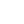# Advent of Code 2022 - Day 5 Javascript Solution

By Henri ParviainenAdvent of Code puzzle of the day had some tricky parsing and it took me a while to figure it out. Here is my Javascipt solution for the day.

## Disclaimer

This solution is not the fastest implementation nor is it claiming to be the best way to solve the problem. There are many ways to solve it and this is just how I did it with Javascript. By sharing I hope it can help someone who is struggling to find a solution to the puzzle.

## Solution

To be able to run my solution, you should have same project setup as me.

First, download the input and save it as a `txt` file in the same directory as you will have your javascript file.

So at the starting point, your folder structure should be:

``````/
-- day5.js
-- input.txt``````

### Parts 1 & 2

Add the following code to your `day5.js` file.

``````let fs = require("fs");

let [inputStacks, inputInstructions] = fs
.toString()
.trim()
.split("\n\n");

let inputStacksLines = inputStacks.split("\n");

// Remove stack numbers line

inputStacksLines.pop();

const stacks = [];

for (let inputStacLine of inputStacksLines) {
for (let i = 0; i < inputStacLine.length; i += 3 + 1) {
let start = i;
let end = start + 3;
let crate = inputStacLine.substring(start, end);

let stackIndex = i / (3 + 1);
if (!stacks[stackIndex]) {
stacks[stackIndex] = [];
}
if (crate.trim()) {
stacks[stackIndex].push(crate.substring(1, 2));
}
}
}

for (let stack of stacks) {
stack.reverse();
}

let instructions = inputInstructions.split("\n").map(line => {
let instruction = line.split(" ");
let amount = parseInt(instruction, 10);
let source = parseInt(instruction, 10) - 1;
let destination = parseInt(instruction, 10) - 1;

return { amount, source, destination };
});

const partOne = () => {
let stacksForPart1 = JSON.parse(JSON.stringify(stacks));
for (let { amount, source, destination } of instructions) {
for (let c = 0; c < amount; c++) {
let crate = stacksForPart1[source].pop();
stacksForPart1[destination].push(crate);
}
}

return stacksForPart1.map(stack => stack[stack.length - 1]).join("");
};

const partTwo = () => {
let stacksForPart2 = JSON.parse(JSON.stringify(stacks));
for (let { amount, source, destination } of instructions) {
let cratesStack = stacksForPart2[source].splice(-1 * amount, amount);
stacksForPart2[destination].push(...cratesStack);
}

return stacksForPart2.map(stack => stack[stack.length - 1]).join("");
};

let topCratesPart1 = partOne();

let topCratesPart2 = partTwo();

console.log("Part one top crates:", topCratesPart1);
console.log("Part two top crates:", topCratesPart2);``````

Run in it with `node day5.js` and you'll have your answers for the day!